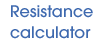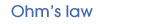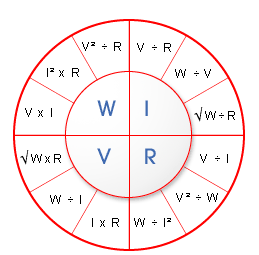##Enter any TWO of the following values, then press the solve button.

Voltage (Volts)
Current (Amps)
Resistance (Ohms)
Power (Watts)“When a current flows through a conductor the current flowing is directly proportional to the potential difference between either end and the resistance of the conductor”.

 R= V ÷ I V² ÷ W W ÷ I² W= V² ÷ R I² × R V × I V= √W × R W ÷ I I × R I= V ÷ R W ÷ V √W ÷ R